New update is available. Click here to update.

# AMCAT Coding Questions

AMCAT Automata is a programming test that can test the coding ability of a student. The syllabus of this includes C, C++, and Java. There are mainly two questions in this test. The first one is based on patterns, and the other is based on strings or matrics.

We will discuss some vital AMCAT Coding Questions asked in the AMCAT coding test. It will help you prepare well for your upcoming AMCAT exam.

## AMCAT Coding Questions

Q1. Write a program to print Floyd’s Triangle.

This is one of the important pattern questions among all AMCAT Coding Questions. And, here is the solution to the above question.

``````#include<stdio.h>
int main()
{
int n, row,  c, a = 1;
printf("Enter the number of rows to print\n");
scanf("%d", &n);
for (row = 1; row <= n; row++)
{
for (c = 1; c <= row; c++)
{
printf("%d ",a);
a++;
}
printf("\n");
}
}``````

Output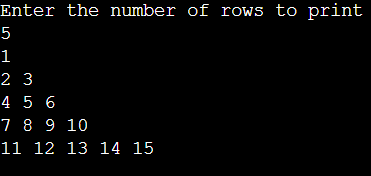Q2. Write a program to print Trapezium Pattern.

``````#include<stdio.h>
int main()
{
int n=4,num=1,i=1,x=0,k=1,number=n;
for(i=0;i<n;i++)
{
for(int j=1;j<=x;j++)
{
printf(" ");
}
for(int m=1;m<2*n-x;m++)
{
if(m%2==0)
printf("%s","*");
else
printf("%d",num++);
}
printf("%s","*");
for(int l=1;l<2*n-x;l++)
{
if(l%2==0)
printf("%s","*");
else
{
printf("%d",k+number*number);
k++;
}
}
number--;

x=x+2;
printf("\n");
}
return 0;
}
``````

Output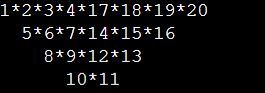Q3. Write a C program to find the GCD of two numbers.

``````#include<stdio.h>
void main()
{
int n1,n2,i,min,GCD;
printf("Enter two numbers: \n");
scanf("%d%d",&n1,&n2);
min = (n1<n2)?n1:n2;
for(i=1;i<=min;i++)
{
if(n1%i ==0 && n2%i==0)
{
GCD = i;
}
}
printf("\nG.C.D of %d and %d is %d", n1, n2, GCD);
}``````

Output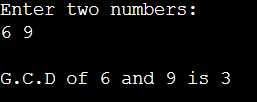Q4. Write a program to print the Fibonacci series in C.

``````#include <stdio.h>
int main() {
int i, n;

// initialize first and second terms
int t1 = 0, t2 = 1;

// initialize the next term (3rd term)
int nextTerm = t1 + t2;

// get no. of terms from the user
printf("Enter the nth term: ");
scanf("%d", &n);

// print the first two values t1 and t2
printf("Fibonacci Series: %d, %d, ", t1, t2);

// print 3rd to nth terms
for (i = 3; i <= n; ++i) {
printf("%d, ", nextTerm);
t1 = t2;
t2 = nextTerm;
nextTerm = t1 + t2;
}

return 0;
}``````

Output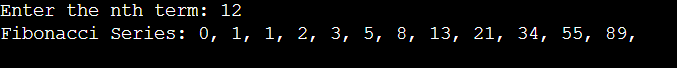Q5. Write a program to check whether a number is Armstrong or not.
This is also an important question in the series of AMCAT Coding Questions. And, here is the solution to the above question.

``````#include <math.h>
#include <stdio.h>

int main() {
int number, originalNum, remainder, n = 0;
float result = 0.0;

printf("Enter an integer: ");
scanf("%d", &number);

originalNum = number;

for (originalNum = number; originalNum != 0; ++n) {
originalNum /= 10;
}

for (originalNum = number; originalNum != 0; originalNum /= 10) {
remainder = originalNum % 10;

result += pow(remainder, n);
}

if ((int)result == number)
printf("%d is an Armstrong number.", number);

else
printf("%d is not an Armstrong number.", number);
return 0;
}
``````

Output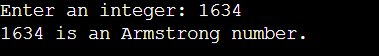Q6. Write a program to reverse a number in C.

``````#include <stdio.h>
int main()
{
int n, rev = 0, remainder;
printf("Enter a number= ");
scanf("%d", &n);
while (n != 0) {
remainder = n % 10;
rev = rev * 10 + remainder;
n /= 10;
}
printf("Reversed number = %d", rev);
return 0;
}
``````

Output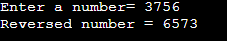Q7. Write a program to create a pyramid using star(*) in C.

``````#include <stdio.h>
int main() {
int i, space, rows, k = 0;
printf("Enter the number of rows: ");
scanf("%d", &rows);
for (i = 1; i <= rows; ++i, k = 0) {
for (space = 1; space <= rows - i; ++space) {
printf("  ");
}
while (k != 2 * i - 1) {
printf("* ");
++k;
}
printf("\n");
}
return 0;
}``````

Output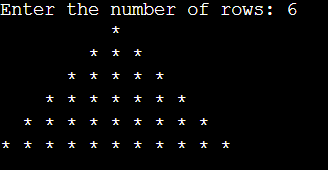Q8. Write a program to remove the duplicate values in a sorted array.

``````#include <stdio.h>
int remove_duplicate(int arr[], int n)
{
if (n == 0 || n == 1)
return n;
int temp[n];

int j = 0;
int i;
for (i = 0; i < n - 1; i++)
if (arr[i] != arr[i + 1])
temp[j++] = arr[i];
temp[j++] = arr[n - 1];

for (i = 0; i < j; i++)
arr[i] = temp[i];

return j;
}

int main()
{
int n;
printf("Enter number of elements in array: ");
scanf("%d", &n);
int arr[n];
int i;
printf("Enter elements of array:\n");
for (i = 0; i < n; i++)
{
scanf("%d", &arr[i]);
}
printf("\nArray Before Removing Duplicates: ");
for (i = 0; i < n; i++)
printf("%d ", arr[i]);

n = remove_duplicate(arr, n);

printf("\nArray After Removing Duplicates: ");
for (i = 0; i < n; i++)
printf("%d ", arr[i]);

return 0;
}``````

Output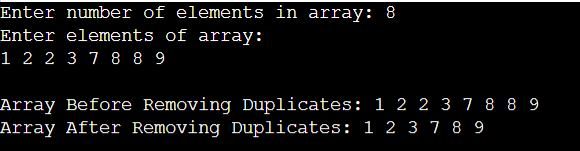Q9. Write a program to find the frequency of a user-given character in a string.
This is one of the tough questions among the listed AMCAT Coding Questions. And, below is the solution to the question.

``````#include <stdio.h>
int main() {
char str, ch;
int count = 0;

fgets(str, sizeof(str), stdin);

printf("Enter a character to find frequency: ");
scanf("%c", &ch);

for (int i = 0; str[i] != '\0'; ++i) {
if (ch == str[i])
++count;
}

printf("Frequency of %c = %d", ch, count);
return 0;
}``````

Output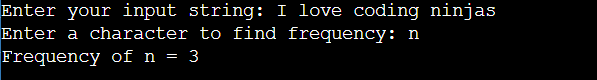Q10. Check whether the given number is prime or not.

``````#include <stdio.h>
int main() {

int n, i, flag = 0;
printf("Enter a positive integer: ");
scanf("%d", &n);
// 0 and 1 are not prime numbers
// change flag to 1 for non-prime number
if (n == 0 || n == 1)
flag = 1;

for (i = 2; i <= n / 2; ++i) {

// if n is divisible by i, then n is not prime
// change flag to 1 for non-prime number
if (n % i == 0) {
flag = 1;
break;
}
}

// flag is 0 for prime numbers
if (flag == 0)
printf("%d is a prime number.", n);
else
printf("%d is not a prime number.", n);

return 0;
}``````

Output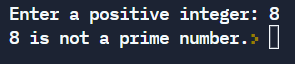Q11. Write a program to print all triplets that form the given sum.

``````#include <stdio.h>
void find_all_triplets(int arr[], int n, int sum)
{
for (int i = 0; i < n - 2; i++) {
for (int j = i + 1; j < n - 1; j++) {
for (int k = j + 1; k < n; k++) {
if (arr[i] + arr[j] + arr[k] == sum) {
printf("%d,%d,%d\n",arr[i],arr[j],arr[k]);
}
}
}
}
}

int main()
{
int n, sum;
printf("\nEnter the number of elements : ");
scanf("%d",&n);
int arr[n];
printf("\nInput the array elements : ");
for(int i = 0; i < n; i++) {
scanf("%d",&arr[i]);
}
printf("\nEnter the sum value : ");
scanf("%d",&sum);
printf("\nThe triplets are \n ");
find_all_triplets(arr, n, sum);
return 0;
}``````

Output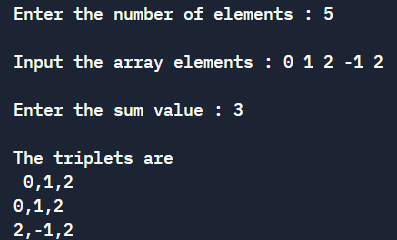Q12. Write a program to find all the roots of a quadratic equation.

``````#include <stdio.h>
#include <math.h>

int main()
{
double a, b, c, discriminant, root1, root2, imaginaryPart, realPart;

printf("Enter coefficients a, b and c: ");
scanf("%lf %lf %lf",&a, &b, &c);

discriminant = b*b-4*a*c;

// condition for real and different roots
if (discriminant > 0) {
// sqrt() function returns square root
root1 = (-b+sqrt(discriminant))/(2*a);
root2 = (-b-sqrt(discriminant))/(2*a);

printf("root1 = %.2lf and root2 = %.2lf",root1 , root2);
}

//condition for real and equal roots
else if (discriminant == 0) {
root1 = root2 = -b/(2*a);

printf("root1 = root2 = %.2lf;", root1);
}

// if roots are not real
else {
realPart = -b/(2*a);
imaginaryPart = sqrt(-discriminant)/(2*a);
printf("root1 = %.2lf+%.2lfi and root2 = %.2f-%.2fi", realPart, imaginaryPart, realPart, imaginaryPart);
}

return 0;
}``````

Output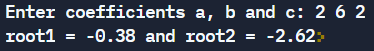Q13. Write a program to find the largest palindrome in an array.

``````#include <stdio.h>

int check_palindrome(int n)
{
int div = 1;
while (n / div >= 10)
div *= 10;

while (n != 0) {
int first = n / div;
int last = n % 10;

if (first != last)
return -1;

n = (n % div) / 10;

div = div / 100;
}
return 1;
}
int large_palindrome(int A[], int n)
{

// Sort the array
for(int i=0; i<=n; i++) {
for(int j=i; j<= n; j++) {
if(A[i] >A [j]) {
int temp = A[i];
A[i] = A[j];
A[j] = temp;
}
}
}

for (int i = n - 1; i >= 0; --i) {
if (check_palindrome(A[i]) == 1)
return A[i];
}
return -1;
}
int main()
{
int a, n, i;
printf("Enter the number of entries: \n");
scanf("%d", &n);
printf("Enter the elements: \n");
for(i=0; i<n; i++)
scanf("%d", &a[i]);
printf("\nLargest Palindrome: %d", large_palindrome(a, n));
return 0;
}``````

Output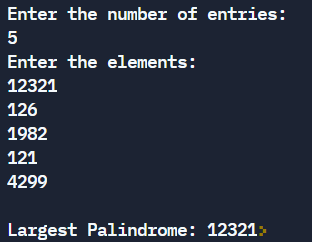Q14. Write a program to convert a number from binary to octal in C.

``````#include <stdio.h>
#include <math.h>

int binary_to_octal(long int binary)
{
int octal = 0, decimal = 0, i = 0;

while(binary != 0) {
decimal += (binary%10) * pow(2,i);
++i;
binary/=10;
}

i = 1;

while (decimal != 0) {
octal += (decimal % 8) * i;
decimal /= 8;
i *= 10;
}

return octal;
}

int main()
{
long int binary;

printf("\nEnter a binary number: ");
scanf("%lld", &binary);

printf("\nOctal Equivalent : %d\n", binary_to_octal(binary));

return 0;
}
``````

Output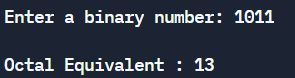Q15. Write a program to print a solid and hollow square star pattern.

``````#include <stdio.h>
int main()
{
int i, j, n;
printf("Enter the size of square: ");
scanf("%d",&n);
for (i = 1; i <= n; i++) {
for (j = 1; j <= n; j++) {
if (i==1 || i==n || j==1 || j==n)
printf("*");
else
printf(" ");
}
printf("\n");
}
return 0;
}
``````

Output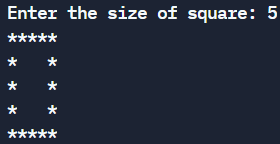## Conclusion

We have discussed the topic of AMCAT Coding Questions. We have seen different types of AMCAT Coding Questions that are asked in the AMCAT exam.

We hope this blog has helped you enhance your knowledge of AMCAT Coding Questions. If you want to learn more, check out our articles JPA Interview QuestionsWPF interview questionsOSPF Interview Questions, and many more on our platform CodeStudio.

But suppose you have just started your learning process and are looking for questions from tech giants like Amazon, Microsoft, Uber, etc. In that case, you must look at the problemsinterview experiences, and interview bundle for placement preparations.

However, you may consider our paid courses to give your career an edge over others!

Happy Learning!